Importance: Medium ✭✭
 Author(s): King, Andrew D. Rabern, L.
 Subject: Graph Theory
 Keywords: independent set maximal clique
 Recomm. for undergrads: yes
 Posted by: Andrew King on: March 31st, 2011
 Solved by:
Conjecture   There is a universal constantsuch that every graph contains a stable set which intersects every maximal clique of size.

Conjecture   Every graph contains a stable set which intersects every maximal clique of size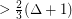.

Both forms of the conjecture are false, as shown by the following counterexample.

Take sufficiently large integersand, and let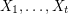be disjoint-cliques, such that their union is a clique of size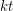. Now adddisjoint copies of, labelled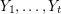, with no edges between them and such thatis complete to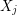if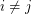and anticomplete otherwise.

This graph has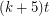vertices and maximum degree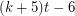. Every edge inis in a maximal clique of size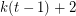. It is straightforward to show that for everywe can chooseandsufficiently large that this graph is a counterexample.

The original discussion of the conjecture follows.

King [K] proved that any graphwithcontains a stable set intersecting every maximum clique. This used the same approach as Rabern's earlier proof for graphs with, but exploited a more general existence condition for independent systems of representatives.

One of the key lemmas is Hajnal's clique collection lemma, which states that for any collection of maximum cliques in a graph, the sum of the intersection and the union is at least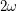. Unfortunately the same result does not hold when restricted to large maximal cliques. Thus the case of maximal cliques would require a different approach.

This conjecture is motivated by a local strengthening of Reed's omega, Delta, chi conjecture.

## Bibliography

[K] Andrew D. King. Hitting all maximum cliques with a stable set using lopsided independent transversals, J. Graph Theory, n/a, doi: 10.1002/jgt.20532.

[R] Landon Rabern. On hitting all maximum cliques with an independent set, J. Graph Theory, 66 (2010), 32--37, doi: 10.1002/jgt.20487.

* indicates original appearance(s) of problem.

## Reply

More information about formatting options